# Trigonometry

Sep. 25, 2014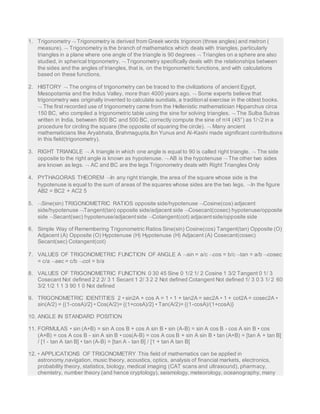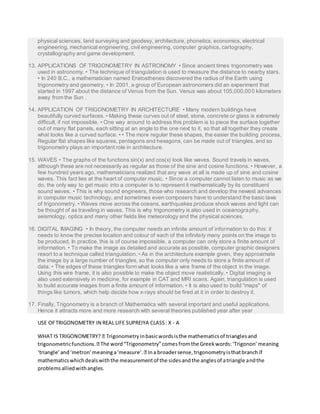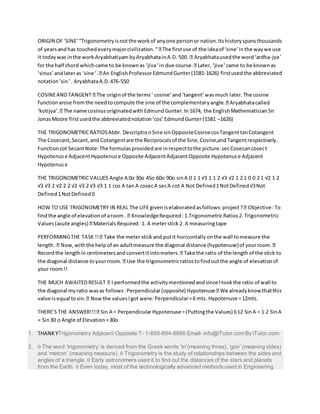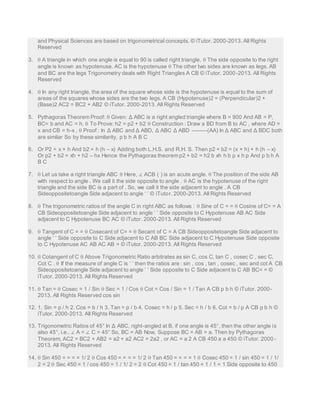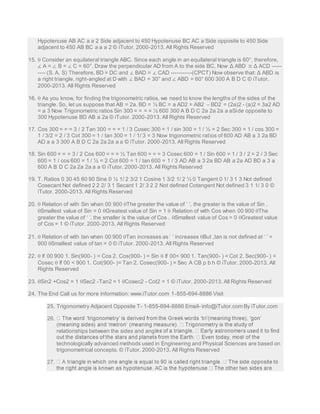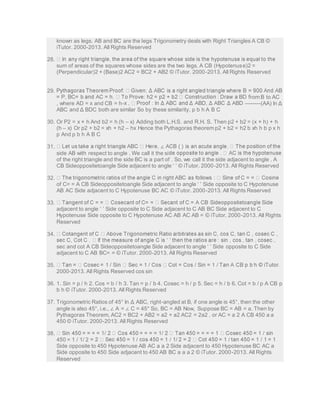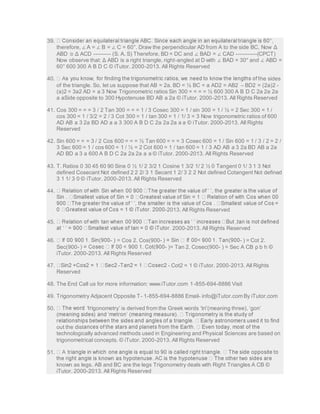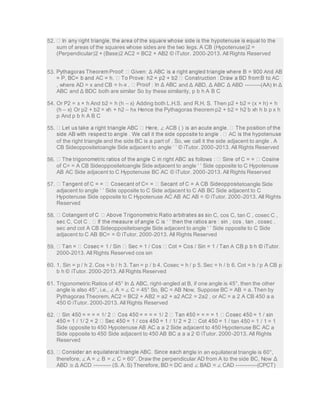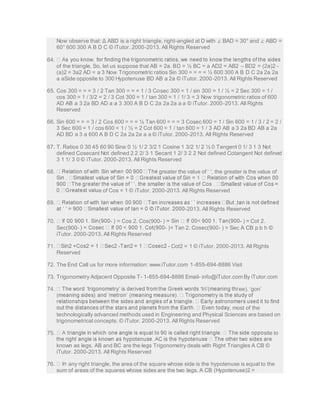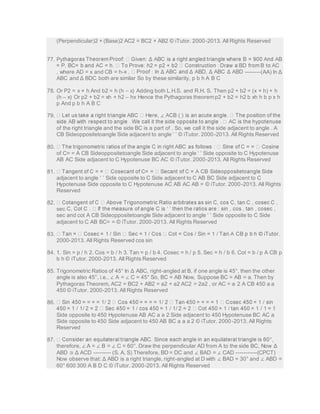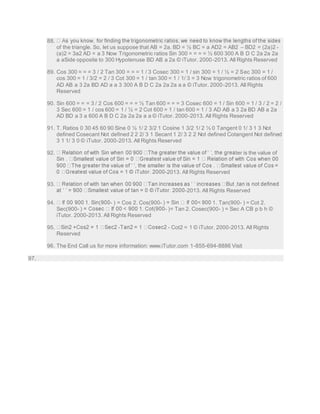Upcoming SlideShare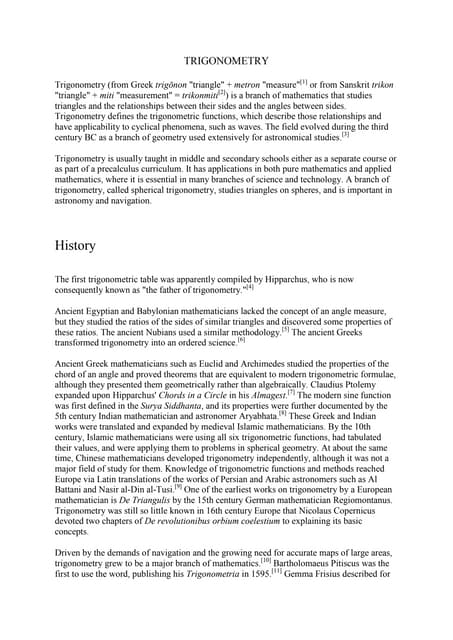Trigonometry
1 of 11

### Trigonometry

1. 1. Trigonometry  Trigonometry is derived from Greek words trigonon (three angles) and metron ( measure).  Trigonometry is the branch of mathematics which deals with triangles, particularly triangles in a plane where one angle of the triangle is 90 degrees  Triangles on a sphere are also studied, in spherical trigonometry.  Trigonometry specifically deals with the relationships between the sides and the angles of triangles, that is, on the trigonometric functions, and with calculations based on these functions. 2. HISTORY  The origins of trigonometry can be traced to the civilizations of ancient Egypt, Mesopotamia and the Indus Valley, more than 4000 years ago.  Some experts believe that trigonometry was originally invented to calculate sundials, a traditional exercise in the oldest books.  The first recorded use of trigonometry came from the Hellenistic mathematician Hipparchus circa 150 BC, who compiled a trigonometric table using the sine for solving triangles.  The Sulba Sutras written in India, between 800 BC and 500 BC, correctly compute the sine of π/4 (45°) as 1/√2 in a procedure for circling the square (the opposite of squaring the circle).  Many ancient mathematicians like Aryabhata, Brahmagupta,Ibn Yunus and Al-Kashi made significant contributions in this field(trigonometry). 3. RIGHT TRIANGLE  A triangle in which one angle is equal to 90 is called right triangle.  The side opposite to the right angle is known as hypotenuse.  AB is the hypotenuse  The other two sides are known as legs.  AC and BC are the legs Trigonometry deals with Right Triangles Only 4. PYTHAGORAS THEOREM In any right triangle, the area of the square whose side is the hypotenuse is equal to the sum of areas of the squares whose sides are the two legs. In the figure AB2 = BC2 + AC2 5 5. Sine(sin) TRIGONOMETRIC RATIOS opposite side/hypotenuse Cosine(cos) adjacent side/hypotenuse Tangent(tan) opposite side/adjacent side Cosecant(cosec) hypotenuse/opposite side Secant(sec) hypotenuse/adjacent side Cotangent(cot) adjacent side/opposite side 6. Simple Way of Remembering Trigonometric Ratios Sine(sin) Cosine(cos) Tangent(tan) Opposite (O) Adjacent (A) Opposite (O) Hypotenuse (H) Hypotenuse (H) Adjacent (A) Cosecant(cosec) Secant(sec) Cotangent(cot) 7. VALUES OF TRIGONOMETRIC FUNCTION OF ANGLE A sin = a/c cos = b/c tan = a/b cosec = c/a sec = c/b cot = b/a 8. VALUES OF TRIGONOMETRIC FUNCTION 0 30 45 Sine 0 1/2 1/ 2 Cosine 1 3/2 Tangent 0 1/ 3 Cosecant Not defined 2 2 2/ 3 1 Secant 1 2/ 3 2 2 Not defined Cotangent Not defined 1/ 3 0 3 1/ 2 60 3/2 1/2 1 1 3 90 1 0 Not defined 9. TRIGONOMETRIC IDENTITIES 2 • sin2A + cos A = 1 • 1 + tan2A = sec2A • 1 + cot2A = cosec2A • sin(A/2) = {(1-cosA)/2} • Cos(A/2)= {(1+cosA)/2} • Tan(A/2)= {(1-cosA)/(1+cosA)} 10. ANGLE IN STANDARD POSITION 11. FORMULAS • sin (A+B) = sin A cos B + cos A sin B • sin (A-B) = sin A cos B - cos A sin B • cos (A+B) = cos A cos B - sin A sin B • cos(A-B) = cos A cos B + sin A sin B • tan (A+B) = [tan A + tan B] / [1 - tan A tan B] • tan (A-B) = [tan A - tan B] / [1 + tan A tan B] 12. • APPLICATIONS OF TRIGONOMETRY This field of mathematics can be applied in astronomy,navigation, music theory, acoustics, optics, analysis of financial markets, electronics, probability theory, statistics, biology, medical imaging (CAT scans and ultrasound), pharmacy, chemistry, number theory (and hence cryptology), seismology, meteorology, oceanography, many
2. physical sciences, land surveying and geodesy, architecture, phonetics, economics, electrical engineering, mechanical engineering, civil engineering, computer graphics, cartography, crystallography and game development. 13. APPLICATIONS OF TRIGONOMETRY IN ASTRONOMY • Since ancient times trigonometry was used in astronomy. • The technique of triangulation is used to measure the distance to nearby stars. • In 240 B.C., a mathematician named Eratosthenes discovered the radius of the Earth using trigonometry and geometry. • In 2001, a group of European astronomers did an experiment that started in 1997 about the distance of Venus from the Sun. Venus was about 105,000,000 kilometers away from the Sun . 14. APPLICATION OF TRIGONOMETRY IN ARCHITECTURE • Many modern buildings have beautifully curved surfaces. • Making these curves out of steel, stone, concrete or glass is extremely difficult, if not impossible. • One way around to address this problem is to piece the surface together out of many flat panels, each sitting at an angle to the one next to it, so that all together they create what looks like a curved surface. • • The more regular these shapes, the easier the building process. Regular flat shapes like squares, pentagons and hexagons, can be made out of triangles, and so trigonometry plays an important role in architecture. 15. WAVES • The graphs of the functions sin(x) and cos(x) look like waves. Sound travels in waves, although these are not necessarily as regular as those of the sine and cosine functions. • However, a few hundred years ago, mathematicians realized that any wave at all is made up of sine and cosine waves. This fact lies at the heart of computer music. • Since a computer cannot listen to music as we do, the only way to get music into a computer is to represent it mathematically by its constituent sound waves. • This is why sound engineers, those who research and develop the newest advances in computer music technology, and sometimes even composers have to understand the basic laws of trigonometry. • Waves move across the oceans, earthquakes produce shock waves and light can be thought of as traveling in waves. This is why trigonometry is also used in oceanography, seismology, optics and many other fields like meteorology and the physical sciences. 16. DIGITAL IMAGING • In theory, the computer needs an infinite amount of information to do this: it needs to know the precise location and colour of each of the infinitely many points on the image to be produced. In practice, this is of course impossible, a computer can only store a finite amount of information. • To make the image as detailed and accurate as possible, computer graphic designers resort to a technique called triangulation. • As in the architecture example given, they approximate the image by a large number of triangles, so the computer only needs to store a finite amount of data. • The edges of these triangles form what looks like a wire frame of the object in the image. Using this wire frame, it is also possible to make the object move realistically. • Digital imaging is also used extensively in medicine, for example in CAT and MRI scans. Again, triangulation is used to build accurate images from a finite amount of information. • It is also used to build "maps" of things like tumors, which help decide how x-rays should be fired at it in order to destroy it. 17. Finally, Trigonometry is a branch of Mathematics with several important and useful applications. Hence it attracts more and more research with several theories published year after year USE OF TRIGONOMETRY IN REAL LIFE SUPREIYA CLASS : X - A he word “Trigonometry” comes from the Greek words: ‘Trigonon’ meaning mathematics which deals with the measurement of the sides and the angles of a triangle and the problems allied with angles.
3. ORIGIN OF ‘SINE’ “Trigonometry is not the work of any one person or nation. Its history spans thousands it toda -jya ’ mund Gunter (1581-1626) first used the abbreviated notation ‘sin ’ . Aryabhata A.D. 476-550 function arose from the need to compute the sine of the complementar Jonas Moore first used the abbreviated notation ‘cos’ Edmund Gunter (1581 –1626) THE TRIGONOMETRIC RATIOS Abbr. Descriptio n Sine sin Opposite Cosine cos Tangent tan Cotangent The Cosecant, Secant, and Cotangent are the Reciprocals of the Sine, Cosine,and Tangent respectively . Function cot Secant Note: The formulas provided are in respect to the picture. sec Cosecan cosec t Hypotenus e Adjacent Hypotenus e Opposite Adjacent Adjacent Opposite Hypotenus e Adjacent Hypotenus e THE TRIGONOMETRIC VALUES Angle A 0o 30o 45o 60o 90o sin A 0 1 1 √3 1 1 2 √3 √2 1 2 1 0 0 2 1 √2 1 2 √3 √3 2 √2 2 2 √2 √3 2 √3 √3 1 1 cos A tan A cosec A sec A cot A Not Defined 1 Not Defined √3 Not Defined 1 Not Defined 0 nometric your room !! Perpendicular = 6 mts. Hypotenuse = 12mts. = Sin 30 o Angle of Elevation = 30o 1. THANK YTrigonometry Adjacent Opposite T- 1-855-694-8886 Email- info@iTutor.com By iTutor.com 2.  The word ‘trigonometry’ is derived from the Greek words ‘tri’(meaning three), ‘gon’ (meaning sides) and ‘metron’ (meaning measure).  Trigonometry is the study of relationships between the sides and angles of a triangle.  Early astronomers used it to find out the distances of the stars and planets from the Earth.  Even today, most of the technologically advanced methods used in Engineering